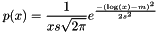#Boost C++ Libraries

...one of the most highly regarded and expertly designed C++ library projects in the world.

## Class template lognormal_distribution

boost::random::lognormal_distribution

## Synopsis

```// In header: <boost/random/lognormal_distribution.hpp>

template<typename RealType = double>
class lognormal_distribution {
public:
// types
typedef normal_distribution< RealType >::input_type input_type;
typedef RealType                                    result_type;

// member classes/structs/unions

class param_type {
public:
// types
typedef lognormal_distribution distribution_type;

// construct/copy/destruct
explicit param_type(RealType = 0.0, RealType = 1.0);

// public member functions
RealType m() const;
RealType s() const;

// friend functions
template<typename CharT, typename Traits>
friend std::basic_ostream< CharT, Traits > &
operator<<(std::basic_ostream< CharT, Traits > &, const param_type &);
template<typename CharT, typename Traits>
friend std::basic_istream< CharT, Traits > &
operator>>(std::basic_istream< CharT, Traits > &, const param_type &);
friend bool operator==(const param_type &, const param_type &);
friend bool operator!=(const param_type &, const param_type &);
};

// construct/copy/destruct
explicit lognormal_distribution(RealType = 0.0, RealType = 1.0);
explicit lognormal_distribution(const param_type &);

// public member functions
RealType m() const;
RealType s() const;
RealType min() const;
RealType max() const;
param_type param() const;
void param(const param_type &);
void reset();
template<typename Engine> result_type operator()(Engine &);
template<typename Engine>
result_type operator()(Engine &, const param_type &);

// friend functions
template<typename CharT, typename Traits>
friend std::basic_ostream< CharT, Traits > &
operator<<(std::basic_ostream< CharT, Traits > &,
const lognormal_distribution &);
template<typename CharT, typename Traits>
friend std::basic_istream< CharT, Traits > &
operator>>(std::basic_istream< CharT, Traits > &,
const lognormal_distribution &);
friend bool operator==(const lognormal_distribution &,
const lognormal_distribution &);
friend bool operator!=(const lognormal_distribution &,
const lognormal_distribution &);
};```

## Description

Instantiations of class template lognormal_distribution model a random distribution . Such a distribution produces random numbers withfor x > 0.

WarningThis distribution has been updated to match the C++ standard. Its behavior has changed from the original boost::lognormal_distribution. A backwards compatible version is provided in namespace boost.

### `lognormal_distribution` public construct/copy/destruct

1. `explicit lognormal_distribution(RealType m = 0.0, RealType s = 1.0);`

Constructs a `lognormal_distribution`. `m` and `s` are the parameters of the distribution.

2. `explicit lognormal_distribution(const param_type & param);`

Constructs a `lognormal_distribution` from its parameters.

### `lognormal_distribution` public member functions

1. `RealType m() const;`

Returns the m parameter of the distribution.

2. `RealType s() const;`

Returns the s parameter of the distribution.

3. `RealType min() const;`

Returns the smallest value that the distribution can produce.

4. `RealType max() const;`

Returns the largest value that the distribution can produce.

5. `param_type param() const;`

Returns the parameters of the distribution.

6. `void param(const param_type & param);`

Sets the parameters of the distribution.

7. `void reset();`

Effects: Subsequent uses of the distribution do not depend on values produced by any engine prior to invoking reset.

8. `template<typename Engine> result_type operator()(Engine & eng);`

Returns a random variate distributed according to the lognormal distribution.

9. ```template<typename Engine>
result_type operator()(Engine & eng, const param_type & param);```

Returns a random variate distributed according to the lognormal distribution with parameters specified by param.

### `lognormal_distribution` friend functions

1. ```template<typename CharT, typename Traits>
friend std::basic_ostream< CharT, Traits > &
operator<<(std::basic_ostream< CharT, Traits > & os,
const lognormal_distribution & ld);```

Writes the distribution to a `std::ostream`.

2. ```template<typename CharT, typename Traits>
friend std::basic_istream< CharT, Traits > &
operator>>(std::basic_istream< CharT, Traits > & is,
const lognormal_distribution & ld);```

Reads the distribution from a `std::istream`.

3. ```friend bool operator==(const lognormal_distribution & lhs,
const lognormal_distribution & rhs);```

Returns true if the two distributions will produce identical sequences of values given equal generators.

4. ```friend bool operator!=(const lognormal_distribution & lhs,
const lognormal_distribution & rhs);```

Returns true if the two distributions may produce different sequences of values given equal generators.